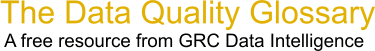Choose Index below for a list of all words and phrases defined in this glossary.

# Regression Coefficient

index | Index

## Regression Coefficient - definition(s)

regression coefficient - [statistics] A value associated with each independent variable in a regression equation, representing the strength and type of relationship the independent variable has to the dependent variable. For example, fire frequency might be modeled as a function of solar radiation, vegetation, precipitation, and aspect. A positive relationship between fire frequency and solar radiation is likely (the more sun, the more frequent the fire incidents). When the relationship is positive, the sign for the associated coefficient is also positive. A negative relationship between fire frequency and precipitation is also likely (places with more rain have fewer fires). Coefficients for negative relationships have negative signs. If the relationship is strong, the absolute value of the coefficient is large. Weak relationships are associated with coefficients near zero.

[Category=Geospatial ]

Source: esri, 30 July 2012 09:45:34, http://support.esri.com/en/knowledgebase/GISDictionary/term/abbreviationData Quality Glossary.  A free resource from GRC Data Intelligence. For comments, questions or feedback: dqglossary@grcdi.nl